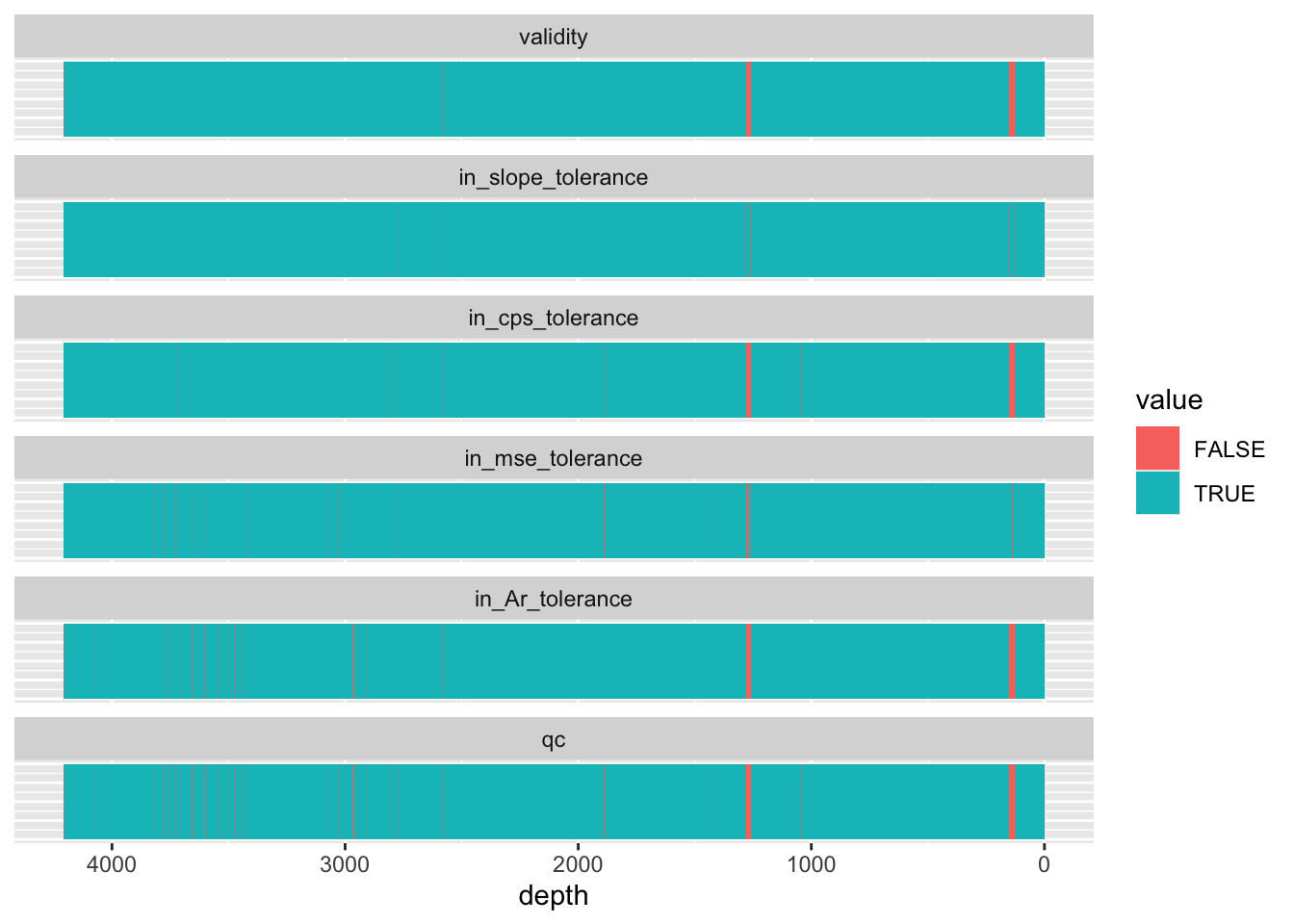## 3.5 Combining “Validity” Flags

It is often desirable to combine all deletion criteria into a single binary variable. This means combining multiple binary variables, returning `FALSE` if any of the values are `FALSE`, but only returning `TRUE` if all of the values are `TRUE`.

``````CD166_19_xrf %>%
select(-any_of("qc")) %>%
mutate(slope = `sample surface` - dplyr::lag(`sample surface`)) %>%
mutate(in_slope_tolerance = ifelse(slope <=-0.1 | slope >=0.1 | is.na(slope) == TRUE, FALSE, TRUE)) %>%
select(-slope) %>%
mutate(in_cps_tolerance = ifelse(cps <=30000 | cps >=60000 | is.na(cps) == TRUE, FALSE, TRUE)) %>%
mutate(in_mse_tolerance = ifelse(MSE <=2, TRUE, FALSE)) %>%
mutate(in_Ar_tolerance = ifelse(Ar >=mean(Ar, na.rm = TRUE) + 2*sd(Ar, na.rm = TRUE) | is.na(Ar), FALSE, TRUE)) %>%
rowwise() %>%
mutate(qc = !any(c(validity, in_slope_tolerance, in_cps_tolerance, in_mse_tolerance, in_Ar_tolerance) == FALSE)) %>%
ungroup() %>%

select(c(depth, validity, in_slope_tolerance, in_cps_tolerance, in_mse_tolerance, in_Ar_tolerance, qc)) %>%
pivot_longer(!depth) %>%
mutate(name = factor(name, levels = c("validity", "in_slope_tolerance", "in_cps_tolerance", "in_mse_tolerance", "in_Ar_tolerance", "qc"))) %>%

ggplot() +
geom_tile(aes(x = depth, y = 1, fill = value)) +
scale_x_reverse() +
facet_wrap(vars(name), ncol = 1) +
theme(axis.title.y = element_blank(),
axis.text.y = element_blank(),
axis.ticks.y = element_blank())``````Bear in mind that this doesn’t remove observations considered defective, only marks them has `qc == FALSE`. If they are to be excluded from subsequent analysis, they should be removed using `filter(qc == TRUE)`.